The benefit of this operator is that it enables changing link properties via other operators of the library Parameters. Additionally, you may use this operator to change other module parameters when a link parameter changes. For that purpose you can define write actions. You can also use this operator to fix link properties and enforce dependencies between several link properties.

In the parameter WriteAction you define what happens when link properties change. The parameter Activate activates the write actions defined in WriteAction:

• If Activate is set to No, then parameters which have the access flag Write may still be changed but no write action is executed. As any link property is a static parameter, the possible write targets must be static, too.
• If the write target is a parameter of the own module, then the addressed module parameter may have the mode Read or Write.
• If the parameter has the mode Read, the write action may be used to enforce certain rules on the link properties.

Write actions are composed of one or several equations. If you define more than one equation, separate the equations via semicolon. On the left-hand side of these equations you define which parameter of which module receives the result of the calculation. Use the notation `this` when referencing a parameter of this module. As soon as the link properties are changed either because of an update of the incoming link or write access to a parameter of this module, the formula(s) on the right-hand side of the equation(s) you defined in parameter WriteAction is/are carried out and the result(s) is/are forwarded to the parameter(s) specified on the left-hand side of the equation(s). As with the left-hand side you can reference parameters of this module using the notation `this`.

Example:

``` \${this.MaxImgWidth} = (\${this.MaxImgWidth} <= 1024) ? \${this.MaxImgWidth} : 1024; ```

The formula can refer to values of static module parameters anywhere in the design. The formula cannot only incorporate the values of parameters, but also specific properties of parameters, such as minimal value, maximal value, step size, or the numerical value of enumeration items.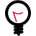Formula syntax: mathematical operations

The syntax complies to the GenICam API standard in version 2.0. The allowed formula elements are identical with the formula elements defined in the GenICam standard:

 Basic operations: () Brackets + - * / Addition, subtraction, multiplication, division % Remainder ** Power & | ^ ~ Bitwise: and / or / xor / not <> = > < <= >= Logical relations: not equal / equal / greater / less / less or equal / greater or equal && || Logical and / logical or << >> Shift left / shift right

Table 41. Basic operations

Conditional operator

<condition> ? <true_expr> : <false_expr>

Example:

\${target.Value} = (\${this.BitWidth} > 8) ? 2 : 1;

 Functions: SGN(x) Returns sign of x. Returns +1 for positive argument and -1 for negative argument. NEG(x) Swaps sign of x. ABS(x) Returns absolute value of x. SQRT(x) Returns square root of x. TRUNC(x) Truncates x, which means returning the nearest integral value that is not larger in magnitude than x. FLOOR(x) Rounds downward, returning the largest integral value that is not greater than x. CEIL(x) Rounds upward, returning the smallest integral value that is not less than x. ROUND(x,precision) Rounds x to the number of decimal fractional digits given by precision, with halfway cases rounded away from zero. SIN(x) Returns sine of an angle of x radians. COS(x) Returns cosine of an angle of x radians. TAN(x) Returns the tangent of an angle of x radians. ASIN(x) Returns the principal value of the arc sine of x, expressed in radians. ACOS(x) Returns the principal value of the arc cosine of x, expressed in radians. ATAN(x) Returns the principal value of the arc tangent of x, expressed in radians. EXP(x) Returns the base-e exponential function of x, which is e raised to the power x: ex. LN(x) Returns the natural logarithm of x. The natural logarithm is the base-e logarithm: the inverse of the natural exponential function (exp). LG(x) Returns the common (base-10) logarithm of x. E() Returns Euler's number, 2.7182818284590451. PI() Returns circle constant, 3.1415926535897931.

Table 42. Functions

Example:

\${target.Value} = NEG(\${this.Parallelism})Paths to parameters

To access an operator parameter any place within your design, you need to provide the path to this parameter in your formula.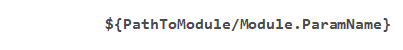`PathToModule`: Here, you define the relative path to the operator whose parameter you want to access. The path is relative to the hierarchical level the translation operator itself is located. You also define the name of the accessed operator. Use a slash as hierarchy separator.

`Module`: Name of the module.

As name for the translation operator instance itself, use the name `this`.

Keep your modules independent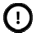It is not allowed to define a path towards a hierarchical level higher than the hierarchical level the translation operator is located at. This rule follows the logic that a hierarchical module is not allowed to know anything about the environment it is instantiated in, because only in this case it can be used as a freely relocatable and replicable module.
 Access to parameter propertiesThe formulas cannot only incorporate the values of parameters, but also specific properties of parameters, such as minimal value, maximal value, or step size: `\${PathToModule/Module.ParamName.From}` or `\${PathToModule/Module.ParamName.Min}`: the minimal valid value of the parameter `PathToModule/Module.ParamName`. `\${PathToModule/Module.ParamName.To}` or ```\${ PathToModule/Module.ParamName.Max}```: the maximum valid value of the parameter `PathToModule/Module.ParamName`. `\${PathToModule/Module.ParamName.Inc}`: increment (step size) between two valid values of the parameter `PathToModule/Module.ParamName`. `\${PathToModule/Module.ParamName.Enum("EnumName")}`: integer value of the enumeration name `EnumName`.
 Syntax for write access equationsYou define the equations for write actions in the parameter WriteAction. They have the following syntax: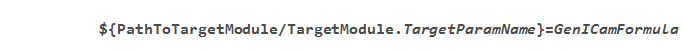Here, you can define multiple equations for multiple target parameters. Use a semicolon as separator between the individual equations. `this` refers to the translation operator instance itself.

You find a general introduction into the library `Parameters` in Library Parameters.

### I/O Properties

Property Value
Operator Type O
Input Link I, Image data input
Output Link O, Image data output

Bit Width [1, 64]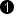As `I`
Arithmetic {Unsigned, signed} As `I`
Parallelism Any As `I`
Kernel Columns Any As `I`
Kernel Rows Any As `I`
Img Protocol {VALT_IMAGE2D, VALT_LINE1D, VALT_PIXEL0D, VALT_SIGNAL} As `I`
Color Format Any As `I`
Color Flavor Any As `I`
Max. Img Width Any As `I`
Max. Img Height Any As `I`
 The range of the input bit width is [1, 64]. For signed inputs, the range is [2, 64]. For unsigned color inputs, the range is [3, 63] and for signed color, the range is [6, 63].

### Parameters

Status
Type static read (although the GUI displays write) parameter
Default None
Range `OK` or an error message occurs

Displays the error status. If the parameter Activate is set to Yes, the other module parameters are checked. This parameter displays the result of this check, i.e., either OK or an error message occurs.

This parameter is not part of the final applet.

Protocol
Type static write parameter
Default VALT_IMAGE2D
Range {VALT_IMAGE2D,VALT_LINE1D,VALT_PIXEL0D,VALT_SIGNAL}

ColorFormat
Type static write parameter
Default VAF_GRAY
Range {VAF_GRAY,VAF_COLOR,VAF_NONE,VAF_UNDEFINED}

ColorFlavor
Type static write parameter
Default FL_NONE
Range {FL_NONE,FL_RGB,FL_HSI,FL_YUV,FL_LAB,FL_XYZ,FL_HSL,FL_HSV,FL_YCrCb}

Allows access to the link property ColorFlavor. Possible values are FL_NONE, FL_RGB, FL_HSI, FL_YUV, FL_LAB, FL_XYZ, FL_HSL, FL_HSV, FL_YCrCb.

Arithmetic
Type static write parameter
Default UNSIGNED
Range {SIGNED, UNSIGNED}

BitWidth
Type static write parameter
Default 1
Range

Parallelism
Type static write parameter
Default 1
Range

KernelRows
Type static write parameter
Default 1
Range

KernelColumns
Type static write parameter
Default 1
Range

MaxImgWidth
Type static write parameter
Default 1024
Range

MaxImgHeight
Type static write parameter
Default 1024
Range

WriteAction
Type static write parameter
Default
Range